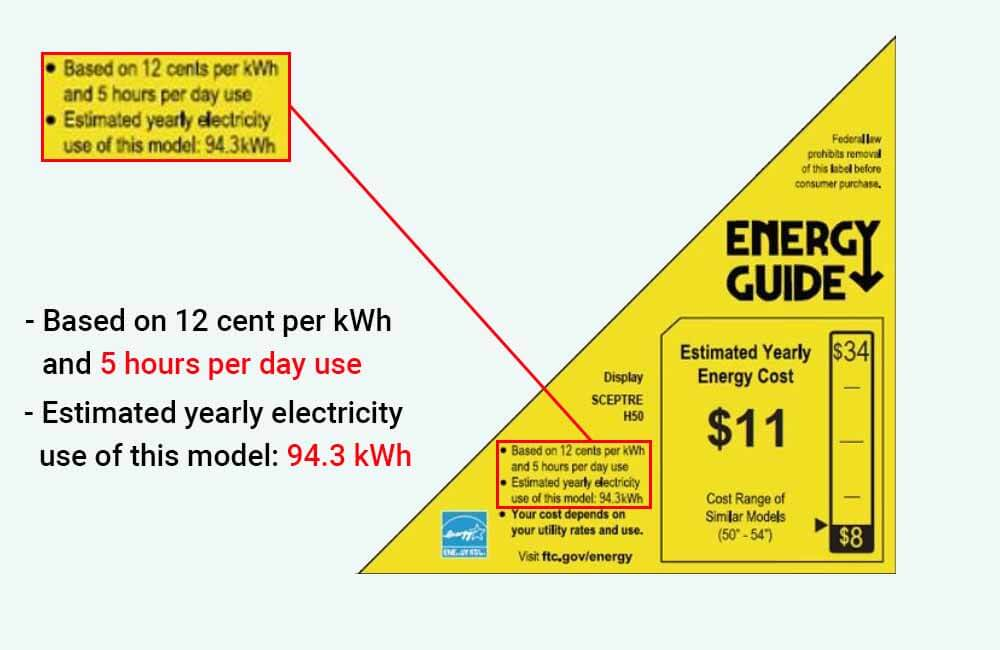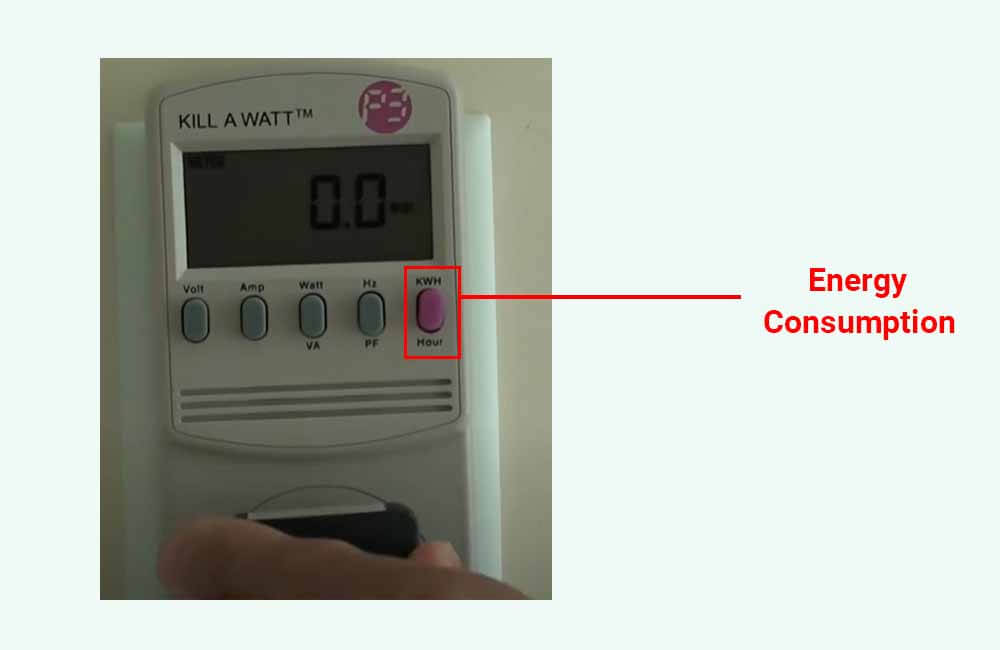# How many watts does a tv use?

There are many types and sizes of TVs, so, if the question is “how many watts does a tv use?”, the answer is “it depends on the TV”.

However, there are a couple of ways to determine the power usage (watts) of your TV, as well as its energy consumption (kWh).

In this article, to provide some perspective, I’ll first list different TV types and sizes and their power usage, and then I’ll show you how to determine the wattage of your TV, and how to calculate (or even measure) its energy consumption (kWh).

## How many watts does a tv use?

The amount of power (watts) that a tv uses can be anywhere from 15 to 250 watts depending on its screen size, type, and model. For example, while a 32″ LED TV uses 35 watts (on average), a 55″ plasma TV can use up to 250 watts of power when it’s on.

To give you an idea, the following table provides different TV sizes and types and their average power usage:

As mentioned above, the figures provided in the table are averages and estimates, and their purpose is to give you some perspective. Newer EnergyStar-rated TVs will generally consume less, however, older TV models are relatively less energy-efficient and will therefore use more power.

The following section explains a couple of methods to accurately determine the power usage (wattage) of YOUR particular TV.

## How many watts does your TV use?

To determine the power usage (wattage) of your TV, you can either:

• Look at the technical specification label stuck to the back of your TV.
• Use the EnergyGuide (yellow & black) label provided by the manufacturer.
• Or use an electricity monitoring device such as the Kill-A-Watt meter.

Let’s look at each of these methods and how they work.

### 1- Using the technical specifications label:

On the back of your TV, you’ll usually find a label that contains things like serial and model numbers. But more importantly, these labels provide the electrical specification of the TV (Voltage, Current, Wattage).

For example, here’s a specifications label from the back of a 32″ Panasonic LCD TV in which the manufacturer specifies 65 Watts as the power usage:When you find the specification label on your TV, look for the “W” (Watts) power rating, and make sure not to confuse it with the “V” rating, which represents the Voltage.

If you can’t find the wattage rating on the label, look for the “A” (Amperage) rating and multiply it by the voltage rating of the TV:

Power (W) = Voltage (V) x Amperage (A)

For example, the following spec label is from a 23″ LED TV from Toshiba:You can see that the manufacturer specifies 0.7 A (Amps) as the amperage of the TV, and 120 V (Volts) as its voltage. Using our equation, we can easily determine the power usage of the TV:

Power (W) = Voltage (V) x Amperage (A)

Power (W) = 120 V x 0.7 A

Power (W) = 84 Watts

However, as specified by the manufacturer, 0.7 Amps is the maximum amount of current that the TV would draw. This means that most of the time, this particular TV is going to use less than 84 Watts of power.

Now if you can’t find these specifications on the label, or can’t find the label, you can use the next method.

### 2- Using EnergyGuide label to determine TV power usage:

All TVs manufactured after May 10, 2011, come with an EnergyGuide label, which estimates the annual energy consumption of the TV and how much it would cost to run it.

For example, the EnergyGuide label shown below is from a 50″ LED TV:The manufacturer estimates that this TV consumes 94.3 kWh (kiloWatt-hours) of energy (not power) per year. This estimate is based on the assumption that the TV will be on for 5 hours per day.

Now, we have 2 relevant pieces of information here:

• The TV runs for 5 hours a day
• And consumes 94.3 kWh (94300 Wh) per year (365 days)

So, how can we use these figures to determine the power usage of the TV?

We’ll simply use the following equation:

Power Usage (W) = Yearly Energy consumption (Wh) ÷ (Daily Run Time (hours) x 365)

Let me explain:

Electrical Energy is measured in Wh (Watt-hours) or kWh (kiloWatt-hours), and its relationship to Electrical Power (Watts) can be expressed as such:

Electrical Energy (Wh) = Electrical Power (W) x Run Time (hours)

or

Electrical Power (W) = Electrical Energy (Wh) ÷ Run Time (hours)

For example, if we know that a TV consumes 100 Wh or 0.1 kWh every 2 hours when it’s on, we can deduce its power usage:

Electrical Power (W) = Electrical Energy (Wh) ÷ Run Time (hours)

Electrical Power (W) = 100 Wh ÷ 2 hours

Electrical Power (W) = 50 Watts

We know that our 50″ LED TV uses 94.3 kWh (94300 Wh) per year and runs for 5 hours a day. First, let’s calculate the energy that the TV consumes each day (or each 5 hours):

Daily Energy Consumption (Wh) = Yearly Energy Consumption (Wh) ÷ 365 days

Daily Energy Consumption (Wh) = 94300 Wh ÷ 365 days

Daily Energy Consumption (Wh) = 258.35 Watt-hours

This means that our TV consumes 258.35 Wh of energy every 5 hours of run-time. The power usage of the TV can be calculated as such:

Electrical Power (W) = Electrical Energy (Wh) ÷ Run Time (hours)

Electrical Power (W) = 258.35 Wh ÷ 5 hours

Electrical Power (W) = 51.67 Watts

According to our calculation, this particular TV uses about 51-52 Watts of power.

If you can’t find the specification sticker or the EnergyGuide label, the last option is to use an electricity monitoring device.

### 3- Using a Kill-A-Watt meter:

Devices such as the Kill-A-Watt meter can display the power usage of your TV and measure its energy consumption over a certain period of time. All you have to do is plug the device into the electrical outlet, and plug your TV into the device.

Here’s an example of how this works:

Now that we know the power usage of your TV, let’s talk about its energy consumption.

## How much energy (kWh) does your TV consume?

The amount of energy (Wh or kWh) that a tv consumes can be anywhere from 15 to 250 Wh/hour depending on its screen size, type, and model. For example, while a 32″ LED TV consumes around 35 watt-hours of energy per hour of use, a 55″ plasma TV can use up to 250 watt-hours (0.25 kWh) per hour.

The following table provides different TV sizes and types and their average hourly energy usage:

To calculate the energy consumption (Wh or kWh) of your TV (hourly, daily, monthly, or yearly), simply multiply the power usage (Watts) of the TV by the amount of time (hours) that it is left on.

For example, let’s say I have a 50″ LED TV that I know uses 60 Watts of power when it’s turned on. Let’s also assume that I’m in the habit of watching TV 4 hours a day.

To calculate the daily energy consumption of the TV, we can use the following formula:

Daily Energy Consumption (Wh) = Power usage (W) x Daily Run time (hours)

Daily Energy Consumption (Wh) = 60 W x 4 hours

Daily Energy Consumption (Wh) = 240 Watt-hours

Daily Energy Consumption (kWh) = 0.24 kiloWatt-hours

Another easy way to estimate how much electricity your tv uses per hour is to use the EnergyGuide label mentioned in the previous section.

And as mentioned above, EnergyGuide labels provide an estimate of the annual electricity usage of a TV assuming it runs for 5 hours a day. So to get an estimate for the hourly energy consumption of the TV, you can use the following formula:

Hourly Energy Consumption (Wh/hour) = Yearly Energy consumption (Wh) ÷ (5 hours x 365)

Hourly Energy Consumption (Wh/hour) = Yearly Energy consumption (Wh) ÷ 1825

For example, the following EnergyGuide label is from a 32″ LED TV:Assuming the TV is on for 5 hours a day, the manufacturer estimates that it would consume 48 kWh or 48000 Wh of energy per year. The amount of electricity the TV uses each hour is:

Hourly Energy Consumption (Wh/hour) = Yearly Energy consumption (Wh) ÷ 1825

Hourly Energy Consumption (Wh/hour) = 48000 Wh ÷ 1825

Hourly Energy Consumption (Wh/hour) = 26.3 Wh/hour

Once you’ve determined how much electricity the TV uses each hour, you can easily calculate its daily, monthly, and yearly energy consumption based on your TV-watching habits.

If estimates are not enough, you can use an electricity monitoring device such as the Kill-A-Watt meter.

All you’ll have to do is:

• Plug the meter into the electrical outlet
• Plug your TV into the meter
• And push the purple button to display energy consumption in kWh (kiloWatt-hours)Once you set it up, it’ll start measuring the energy consumption of the TV for as much time as you need.